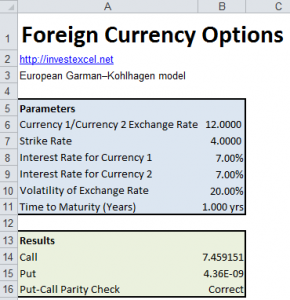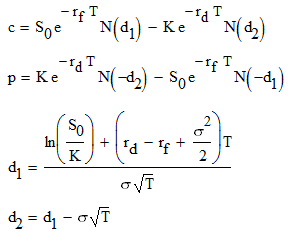# Pricing Foreign Exchange Options

Foreign exchange options (also known as foreign currency options) help investors hedge against exchange rate fluctuations.  They  give the purchaser the right to exchange one currency for another at a fixed price.

At expiry, if the prevailing market exchange rate is better value than the strike rate, the option is out of the money and is usually not exercised. If the option is in the money, then the option is usually exercised (and the cost of the option is partially offset by the more favorable exchange rate)

The Garman-Kohlhagen model was developed in 1983 and is used to price European style foreign currency options.  Prices of foreign exchange options are often given in terms of their implied volatilities, as calculated by the Garman-Kohlhagen modelThe Garman-Kohlhagen model is similar to the model developed by Merton to price options on dividend-paying stocks, but allows borrowing and lending to occur at different rates. Additionally, the underlying exchange rate is assumed to follow Geometric Brownian Motion, and the option can only be exercised at maturity.

The equations are•  rd and rf are the domestic and foreign interest rates
• S0 is the spot rate (i.e. foreign exchange rate)
• K is the strike
• T is the maturity time
• σ is the foreign exchange rate volatility
• N is the cumulative normal distribution

This spreadsheet uses these equations to calculate the price of a foreign currency option. Moreover, the spreadsheet also calculates if put-call parity is satisfied.